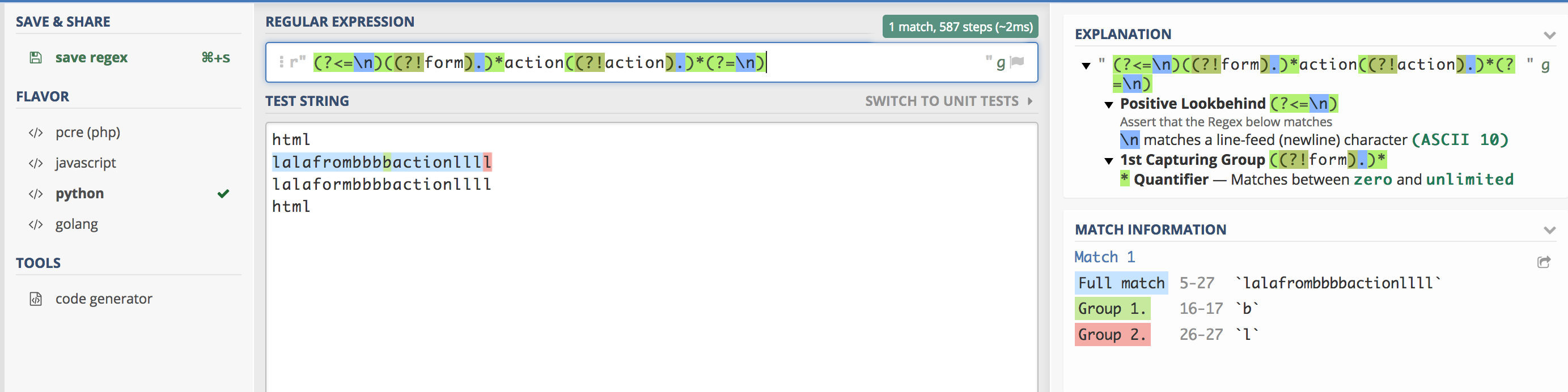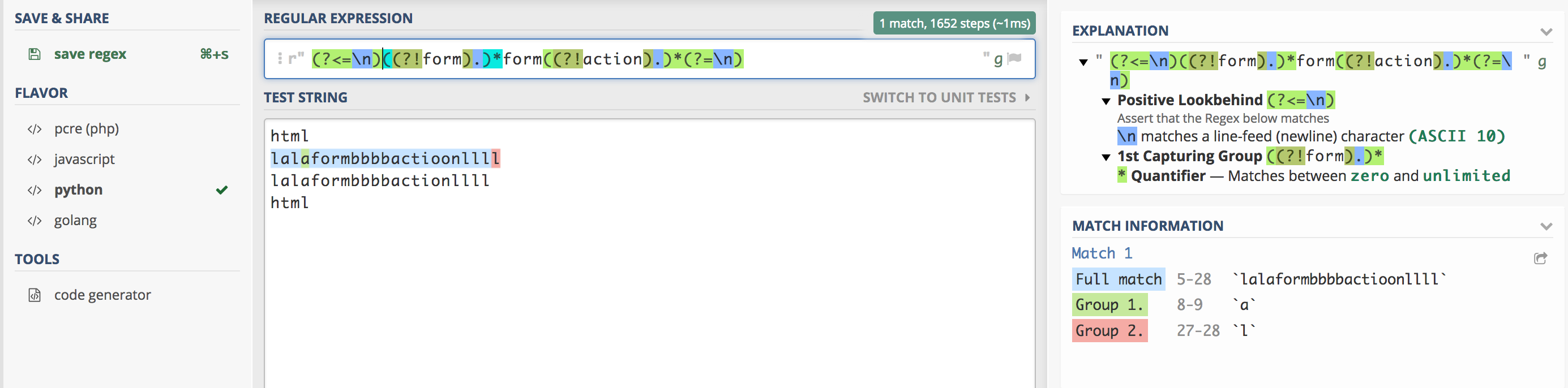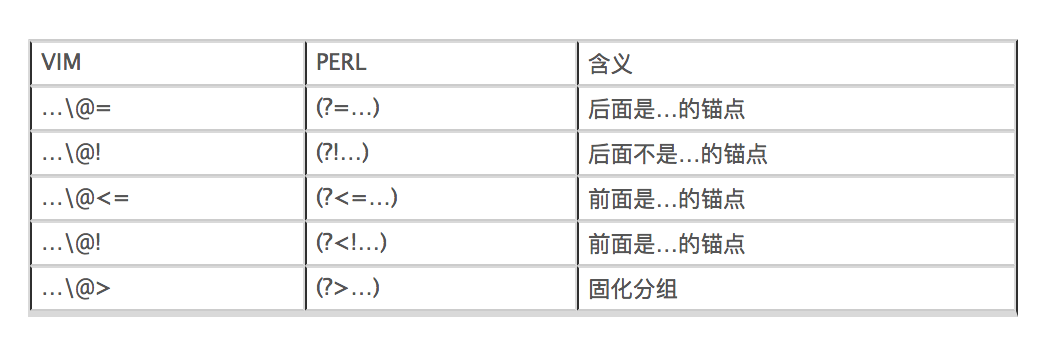# 四种环视匹配

### 0x01 detail

``````肯定顺序环视(?=...)     positive lookahead  (中文也有翻译成:后向界定)

``````

``````是      =

``````

### 0x02 example

#### a.前面没有form后面有action上图共使用了`(?<=)`,`(?!=)`,`(?=)`,没有用到`(?<!)`

#### b.前面有form后面没有action上图共使用了`(?<=)`,`(?!)`,`(?=)`,没有用到`(?<!)`

### 0x03 vim中的环视匹配注意

``````1.其中上图的倒数第2个应该是:`...\@<!`
2.匹配时是正常匹配,不是\v(very magic)模式匹配
``````

### 0x04 attention

1.为了匹配到一个字符串变量中没有form,可以有4种方法:

a)`.(?<!form)`对应的`(.(?<!form))*`
b)`(?<!form).`对应的`((?<!form).)*`
c)`.(?!form)`对应的`(.(?!form))*`
d)`(?!form).`对应的`((?!form).)*`

2.四种环视匹配`(...)`匹配的结果是一个隐藏的位置,是一个位置,不是多个位置,也不是字符

3.`"ifconfig | ack '(?<=inet )(.*)(?= netmask)' -o"`可得到ip地址
`echo lllnihaolllnihaoxxxnihao666 | ack -o '(?<=x)(?=n)nihao.*'`其中的`(?<=x)(?=n)`两个紧挨的环视匹配得到的是一个 隐藏的位置,因为环视匹配不会匹配到字符(不捕获),于是第1个环视匹配得到1个位置后由于无法前进字符串,所以第2个环视匹配 得到的依然是相同的位置处,同理,多个紧挨的环视匹配得到的是相同的位置,说明多个环视匹配紧挨时,匹配结果与各个环视匹配 表达式的先后顺序无关